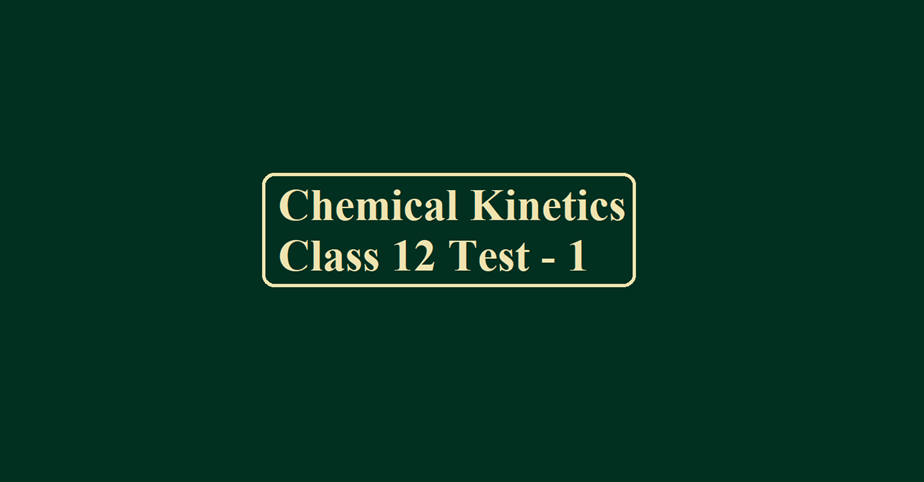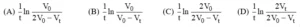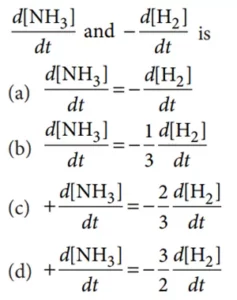# Chemical Kinetics Class 12 Test – 1# Chemical Kinetics Class 12 Test – 1

Chemical Kinetics

Que 1. The decomposition of phosphine (PH3) on tungsten at low pressure is a first-order reaction. It is because the
(A) Rate is proportional to the surface coverage
(B) Rate is inversely proportional to the surface coverage
(C) Rate is independent of the surface coverage
(D) Rate of decomposition is very slow

Que 2. For the first-order reaction, A (g) → B (g) + C (g) + D (s) taking place at constant pressure and temperature condition. Initially, the volume of the container containing only A was found to be V0 and after time ‘t’ it was Vt. Rate constant for the reaction isQue 3. The rate law for the dimerization of NO2 is  = k [NO2]2. Which of the following changes will change the value of the specific rate constant, k;
(A) Doubling the total pressure on the system
(B) Doubling the temperature
(C) Both
(D) None

Que 4. According to the Arrhenius equation, a straight line is to be obtained by plotting the logarithm of the rate constant of a chemical reaction (log k) against –
(A) T
(B) log T
(C) 1/T
(D) log (1/T)

Que 5. The reaction A → B follows first-order kinetics. The time taken for 0.8 moles of A to produce 0.6 moles of B is 1 hour. What is the time taken for the conversion of 0.9 moles of A to produce 0.675 moles of B?
(A) 1 hour
(B) 0.5 hour
(C) 0.25 hour
(D) 2 hours

Que 6. The rate of a reaction increases 4-fold when the concentration of reactant is increased 16 times. If the rate of reaction is 4 × 10–6 mole L–1 S–1 mole L–1 when the concentration of the reactant is 4 × 10–4, the rate constant of the reaction will be
(A) 2 × 10–4 mole1/2 L–1/2 S–1
(B)1 × 10–2 S–1
(C) 2 × 10–4 mole–1/2, L1/2 S–1
(D) 25 mole–1 L min–1

Que 7. The rate of the simple reaction 2 NO + O2 → 2 NO2 when the volume of the reaction vessel is doubled–
(A) Will grow eight times its initial rate
(B) Rates reduced to one-eights of their initial rate
(C) Will grow four times of its initial rate
(D) Reduce to one-fourth of its initial rate

Que 8. 2A → B + C, it would be a zero-order reaction when
(A) the rate of reaction is proportional to the square of the concentration of A
(B) the rate of reaction remains the same at any concentration of A
(C) the rate remains unchanged at any concentration of B and C
(D) the rate of reaction doubles if the concentration of B is increased to double.

Que 9. For the reaction, H2(g) + Br2(g) → 2 HBr(g), the reaction rate = K[H2][Br2]1/2. Which statement is true about this reaction?
(A) The reaction is of second-order
(B) Molecularity of the reaction is 3/2
(C) The unit of K is sec–1
(D) Molecularity of the reaction is 2.

Que 10. The first-order rate constant for the decomposition of dimethyl ether is 3.2 × 10–4s–1 at 450°C.
(CH3)2O(g) → CH4(g) + H2(g) + CO(g) The reaction is carried out in a constant volume flask.  Initially, only dimethyl ether is present, and the pressure is 0.350 atm.  What is the pressure of the system after 8 minutes? (Assume ideal behavior). [Antilog 0.0667 = 1.166]
(A) 0.35 atm
(B) 0.40 atm
(C) 0.45 atm
(D) 0.50 atm

Chemical Kinetics

Que 11. When a biochemical reaction is carried out in the laboratory, outside the human body in absence of an enzyme, the rate of reaction obtained is 10–6 times, and the activation energy of a reaction in the presence of an enzyme is
(A) 6/RT
(B) P is required
(C) Different from Ea obtained in the laboratory
(D) Can’t say anything.

Que 12. The chemical reaction, 2O3 → 3O2 proceeds as follows:
O3 → O2 + O                   …… (fast)        O + O3 → 2O2            …… (slow)
The rate law expression should be:
(A) r = K [O3]2
(B) r = K[O3]2 [O2]–1
(C) r = K [O3] [O2]
(D) Unpredictable

Que 13. The time required to decompose half of the substance for the nth-order reaction is inversely proportional to:
(A) an+1
(B) an–1
(C) an–2
(D) an

Que 14. In which rate law is the overall reaction order equal to 3?
(A) Rate = k [A]2[B]0
(B) Rate = k [A]2[B]–1
(C) Rate = k [A]1[B]3
(D) Rate = k [A]3[B]0

Que 15. For the elementary step,  (CH3)3.CBr (aq)  → (CH3)3 C+(aq) + Br¯ (aq) the molecularity is:
(A) Zero
(B) 1
(C) 2
(D) cannot ascertained

Que 16. For a reaction for which the activation energies of the forward and reverse directions are equal in value:
(A) Stoichiometry is the mechanism
(B) ΔH = 0
(C) ΔS = 0
(D) the order is zero.

Que 17. Which statement is not true for the reaction? C + D → F + G if the rate law is rate = k[C]2[D]1/2?
(A) If the concentration of D is increased by a factor of 4, then the reaction rate will double.
(B) If the concentration of C is doubled and the concentration of D is tripled, then the reaction rate will increase by a factor of 6.9.
(C) If the concentration of C is increased by a factor of 3 and the concentration of D is decreased by a factor of 4, then the reaction rate will increase by a factor of 4.5.
(D) If the concentrations of C and D are doubled, then the reaction rate will double.

Que 18. The rate of reaction triples when the temperature changes from 20ºC to 50ºC. Calculate the energy of activation for the reaction. [ln 3 = 1.098]
(A) 28.81 kJ mol–1
(B) 38.51 kJ mol–1
(C) 18.81 kJ mol–1
(D) 8.31 kJ mol–1

Que 19. Which statement is true regarding the experimental determination of a rate law?
(A) In order to determine the value of an exponent for a reactant in the rate law, the concentration of that reactant and one other reactant must be changed.
(B) The instantaneous rate at the midway point of the reaction is used to determine the exponents and rate law for that equation.
(C) The average rate of reaction at the midway point of the reaction is used to determine the exponents and rate law for that equation.
(D) Initial rates of reaction are used to determine the exponents and rate law for that equation.

Chemical Kinetics

Que 20. The reaction CO(g) + Cl2(g) → COCl2(g) is first order with respect to CO, and 3/2 order with respect to Cl2. If the units for rate are M/min, what are the units of the rate constant in this reaction?
(A) min M3/2
(B) 1 / (min M3/2)
(C) 1/ (min M5/2)
(D) 1 / (min M7/2)

Que 21. Which situation is not characteristic of the collision theory model of the reaction A + BC → AB + C?
(A) The electron density around A, B, and C must change.
(B) The orientation of the two species must be correct.
(C) As A approaches BC, a strong attraction between any filled shells of electrons will develop between the two species.
(D) The kinetic energy of the molecules may be the only source of energy for attaining the activation energy. The collisions of A and BC that result in a reaction are a very small fraction of the total number of collisions.

Que 22. Consider the reaction : N2(g) + 3H2(g) → 2NH3(g). The equality relationship betweenQue 23. An increase in the concentration of the reactants of a reaction leads to a change in
(A) Activation energy
(B) Heat of reaction
(C) Threshold energy
(D) Collision frequency

Que 24. If the rate of the reaction is equal to the rate constant, the order of the reaction is
(A) 0
(B) 1
(C) 2
(D) 3

Que 25. When the initial concentration of the reactant is doubled, the half-life period of a zero-order reaction
(A) Is halved
(B) Is doubled
(C) Is tripled
(D) Remains unchanged.

Chemical Kinetics

Que 26. By the action of enzymes, the rate of biochemical reaction
(A) Does, not change
(B) Increases
(C) Decreases
(D) Either (a) or (c).

Que 27. If a reaction A + B → C is exothermic to the extent of 30 kJ/mol and the forward reaction has an activation energy of 70 kJ/mol, the activation energy (in kJ/mol) for the reverse reaction is

Que 28. The rate of the reaction: A + B + C → Product is given by rate =d[A]/dt = k[A]1/2 [B]1/4 [C]0. The order of the reaction is (X/4). Find the value of X.

Que 29. If the rate expression for the reaction 5 Br + BrO3 + 6 H+ → 3 Br2 + 3 H2O; is K[Br][BrO3][H+]2 the order of reaction is

Que 30. The elementary step of the reaction, 2Na + Cl2→ 2NaCl is found to follow 3rd order kinetics, its molecularity is

Chemical Kinetics

# (Solutions) Chemical Kinetics Class 12 Test – 1

For more visit Sciencemotive.com